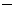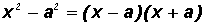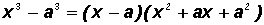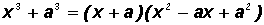A REVIEW OF FACTORINGDefinition of Factoring

Factoring actually means to "divide out".  When you are asked to "factor" a polynomial expression, you are actually told to write the expression as a product of factors.  Often you are asked to factor "relative to the integers."  This means that your factors cannot include rational, irrational, or imaginary numbers.

Some Factoring Methods

A.   Factor out the Greatest Common Factor.

• Find the greatest common numeric factor of all of the coefficients.
• Find the greatest common factor of all of the variables.
• Divide out the greatest common factor

Example 1:

Given the polynomial expression 3x718x3 + 9x2 , factor out the Greatest Common Factor.

We can easily find the greatest common numeric factor by "casual observation."  It is 3.  The greatest common variable factor is x 2 .

In summary, 3x718x  + 9x2 = 3x2(x 56x + 3).

Example 2:

Given the polynomial expression 8x2 + 4, factor out the Greatest Common Factor.

We can easily find the greatest common numeric factor by "casual observation."  It is 4.  There is no greatest common variable factor.

In summary, 8x2 + 4 = 4(2x2 + 1).

Example 3:

Given the polynomial expression x(x3)(x3), factor out the Greatest Common Factor.

This expression has two terms and both contain the factor x3.  This is the Greatest Common Factor and we will factor it out as follows:

2x(x3)(x3) = (x3)( 2x1)

Please note that "factoring" means to "divide out".  When we divide x3 out of the first term, we are left with a factor of 2x; and when we divide x3 out of the second term we are left with a factor of1.

B.   Factor relative to the integers using the Grouping Method.

• Collect the terms in an expression into two groups.
• Factor out the greatest common factor from each group.  You should end up with an identical multinomial factor in each term.  If not, regroup, then factor out the greatest common factor again.  If you still don't end up with an identical multinomial factor in each term, the expression is NOT factorable by grouping.
• Factor out the identical multinomial factor from each term.

Example 4:

Try to factor the polynomial expression x34x2 + 2x8 relative to the integers using the Grouping Method.

Let's form two groups as follows:  (x34x2) + (2x8)

Please note that these were the most convenient groups. It just so happens that when we factor common factors out of both, we have an identical binomial factor in each term!

x2 (x4) + 2(x4) Note that we now have an identical binomial factor in each term!

Lastly, we will factor out the identical binomial factor (x4) as follows:

(x4)(x2 + 2)

In summary, x34x2 + 2x8 = (x4)(x2 + 2).

C.   Factoring the Trinomial ax 2 + bx + c relative to the Integers.

• Find two integers whose product equals ac and whose sum equals b. These integers are always factors of ac. If this is not possible, the trinomial cannot be factored relative to the integers.
• Replace the coefficient b of the middle term bx with the sum of the integers and distribute the variable.
• Use the factoring by grouping method to find a product of two factors.

Example 5:

Try to factor the trinomial 2x2 + 7x + 6 relative to the integers.

Given the general trinomial ax2 + bx + c, we find that a = 2, b = 7, and c = 6, and ac = 2(6) = 12.

We need to find two integers whose product equals 12 and whose sum equals 7.  However, the guessing is simplified because the integers MUST be factors of 12

Let's write down the factor pairs of 12:1,122,63,4     1, 12     2, 6     3, 4

It seems that the two integers we need are 3 and 4 since 3(4) = 12 and 3 + 4 = 7.

Now we write 2x2 + 7x + 6 as follows:

2x2 + 3x + 4x + 6

Next, we'll use the factoring by grouping method to get (2x2 + 3x) + (4x + 6).

Then, we factor the common factor out of both groups as follows:

x(2x + 3) + 2(2x + 3)

Lastly, we will factor out the identical binomial factor to get (2x + 3)(x + 2).

In summary, 2x2 + 7x + 6 = (2x + 3)(x + 2)

As a beginner you might want to use FOIL to convince yourself that you have indeed found the correct factors.

Example 6:

Try to factor the trinomial 6x2 + x2 relative to the integers.

Given the general trinomial ax2 + bx + c, we find that a = 6, b = 1, and c =2, and ac = 6(2) =12.

We need to find two integers whose product equals12 and whose sum equals 1

However, the guessing is simplified because the integers MUST be factors of12.

Let's write down the factor pairs of12:1, 122, 63, 4     1,12     2,6     3,4

It seems that the two integers we need are3 and 4 since3(4) =12 and3 + 4 = 1.

Now we write 6x2 + x2 as follows:

6x23x + 4x2

Next, we'll use the factoring by grouping method to get (6x23x) + (4x2).

Then, we factor the common factor out of both groups as follows:

3x(2x1) + 2(2x1)

Lastly, we will factor out the identical binomial factor to get (2x1)(3x + 2).

In summary, 6x2 + x2 = (2x1)(3x + 2)

As a beginner you might want to use FOIL to convince yourself that you have indeed found the correct factors.

Example 7:

Try to factor the trinomial x25x + 6 relative to the integers.

Given the general trinomial ax2 + bx + c, we find that a = 1, b =5, and c = 6, and ac = 1(6) = 6.

We need to find two integers whose product equals6 and whose sum equals5

However, the guessing is simplified because the integers MUST be factors of 6.

Let's write down the factor pairs of 66,12,3     2, 3     1, 6

It seems that the two integers we need are2 and3 since2(3) = 6 and2 + (3) =5.

Now we write x25x + 6 as follows:

x22x3x + 6

Next, we'll use the factoring by grouping method to get (x22x) + (3x + 6).

Then, we factor the common factor out of both groups as follows:

x(x2)3(x2)

Lastly, we will factor out the identical binomial factor to get (x2)(x3).

In summary, x25x + 6 = (x2)(x3)

As a beginner you might want to use FOIL to convince yourself that you have indeed found the correct factors.

D.   Factoring "special" polynomials relative to the Integers.

• Difference of Squares:• Difference of Cubes:• Sum of Cubes:Please note that a Sums of Squares cannot be factored relative to the integers.  The factors of Sums of Squares are imaginary!  This will be discussed at a later time!

Example 8:

Factor the following "special" polynomials relative to the integers.

(a)   x29

Using the Difference of Squares formula with a = 3 and a2 = 9, we can factor as follows:

(x3)(x + 3)

(b)    x38

Using the Difference of Cubes formula with a = 2 and a2 = 4, we can factor as follows:

(x2)(x2 + 2x + 4)

(c)   x3 + 125

Using the Sum of Cubes formula a = 5,and a2 = 25, we can factor as follows:

(x + 5)(x25x + 25)